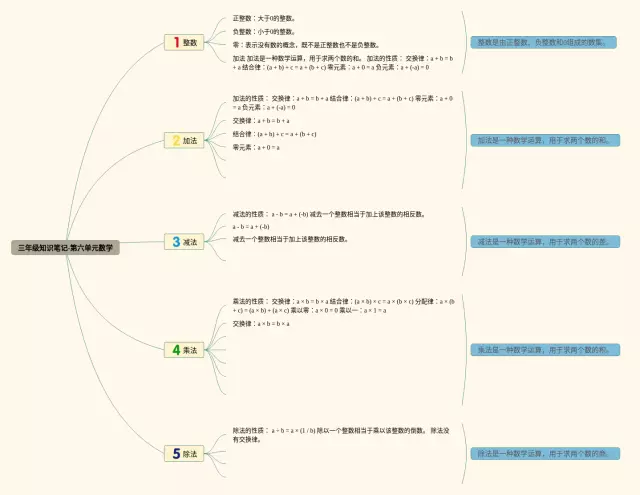# 第六单元思维导图数学三年级(3个可下载)## 思维导图知识点说明：

1. 整数:正整数：大于0的整数。
2. 加法:加法的性质： 交换律：a + b = b + a 结合律：(a + b) + c = a + (b + c) 零元素：a + 0 = a 负元素：a + (-a) = 0
3. 减法:减法的性质： a - b = a + (-b) 减去一个整数相当于加上该整数的相反数。
4. 乘法:乘法的性质： 交换律：a × b = b × a 结合律：(a × b) × c = a × (b × c) 分配律：a × (b + c) = (a × b) + (a × c) 乘以零：a × 0 = 0 乘以a × 1 = a
5. 除法:除法的性质： a ÷ b = a × (1 / b) 除以一个整数相当于乘以该整数的倒数。除法没有交换律。

Tags： 导图 三年级 数学 思维 下载